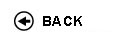Politecnico di Torino
Academic Year 2017/18
06LSLLM, 06LSLOA
Automatic control
1st degree and Bachelor-level of the Bologna process in Computer Engineering - Torino
 Teacher Status SSD Les Ex Lab Tut Years teaching Canale MassimoAC ING-INF/04 79 0 21 0 10
 SSD CFU Activities Area context ING-INF/04 10 B - Caratterizzanti Ingegneria dell'automazione
 Subject fundamentals The course is taught in English. The course will address the fundamentals of dynamical systems analysis and of the design of simple analogic and digital feedback controllers. Expected learning outcomes By the end of this course, students will gain the following knowledge and skill: - Knowledge of the concept of dynamical system together with its mathematical representations such as state equations and transfer functions - Skill in deriving mathematical models of linear dynamical systems - Skill in computing the solution of the system state equations - To evaluate the behaviour of a dynamical system through numeric simulation - Knowledge of structural properties (stability, reachability, observability) of dynamical systemes - Skill in studying the structural properties - Knowledge of the concept of feedback control of dynamical systems - Knowledge of the main performance requirements of feedback systems - Knowledge of the main feedback system analysis techniques based on sinusoidal tools - Skill in analyzing stability and performance of feedback control systems - Knowledge of the design techniques of feedback controllers based on lead and lag functions - Skill in designing feedback controllers for single input single output systems through lead, lag and PID functions - Knowledge of sampled data control systems and realization through digital filters - Skill in designing sampled data control systems - To evaluate the behaviour and performance of controlled systems through numerical simulation Prerequisites / Assumed knowledge Requirements: differential and integral calculus of vector valued real functions, basic concepts of physics (mechanics, electric circuits, ...), complex numbers, complex functions, Laplace transform, real rational functions, linear algebra, basic skill of Matlab. Signal and sampling theory. Z-transform. Contents -Introduction to dynamical systems. State space representation. Examples of state space representation of physical systems. (10 hr) -Solution of state equations, modal analysis and stability of linear systems. Transfer function. (15 hr) - Minimality, reachability and observability, realization. (5 hr) - Nonlinear dynamical systems. Equilibrium solution, linearization around an equilibrium solution, stability of equilibrium solutions through the linearization approach. (10 hr) - Introduction to Output feedback control. Block algebra. (5 hr) - Bode, polar, Nyquist and Nichols diagrams. Nyquist stability criterion. Stability margins (15 hr) - Feedback systems response in face of polynomial inputs; steady state tracking errors, disturbance attenuation and rejection. Time and frequency response of first and second order systems. Feedback systems performance: transient and steady state. (10 hr) - Control design by means of sinusoidal tools and using lead, lag and PID functions (15 hr) - Analysis and design of sampled data control systems. (10 hr) - Short accounts of control design in state space. (5 hr) Delivery modes Theoretical and methodological lessons will be delivered together with example developments by face-to-face instruction in the classroom. Computer laboratory activities are aimed at developing the student�s skill through proper training. Each student is supposed to practice individually with the aid of laboratory work stations. The primary purpose of the laboratory exercises is to apply the methodologies presented in class, through the use of MatLab and Simulink. During the last week of the course, an exam simulation in the laboratory will be offered. Texts, readings, handouts and other learning resources The main reference textbooks are: N. S. Nise: "Control System Engineering", 5th Edition, Wiley, 2008. R. C. Dorf, R. H. Bishop: "Modern Control Systems", 10th Edition, Prentice Hall, 2005. G. F. Franklin, J.D. Powell, A. Emami-Naeini, "Feedback Control of Dynamic Systems", 5th Edition, Prentice Hall, 2006. K. Ogata, "Modern Control Engineering", 4th Edition, Prentice Hall, 2002. P. Bolzern, R. Scattolini, N. Schiavoni, Fondamenti di controlli automatici, Ed. McGraw-Hill Libri Italia, Milano, 3a edizione, 2008 G. Calafiore, Elementi di Automatica, CLUT, Torino, 2007, II ediz. G. Calafiore, Appunti di Controlli Automatici, CLUT, Torino, 2006. A. Isidori, Sistemi di Controllo � Vol. Primo, Ediz. Scientifiche Siderea, Roma, 1992. II ediz. Lecture slides will be available on "Portale della didattica" as well as laboratory practice handouts. Assessment and grading criteria Written exam in computer laboratory lasting 3 hours divided into three parts: Part I. 3 multiple choice problems for each 4 possible answers are shown, only one of which is correct (maximum score: 9/30). Exact answer: 3 points, wrong answer: -1 point, missing answer: 0 points. Comments on the solution must be provided too: in the absence of any comments a null score is given even in the presence of the correct answer. The goal of this first part of the exam is to verify the understanding of the fundamental theoretical topics of analysis and design of feedback control systems. Part II. 1 "open" question on conceptual topics (maximum score: 9/30). The goal of this part of the exam is to verify the student ability to properly express and elaborate either theoretical issues or conceptual problems. Part III. 1 control design problem (maximum score: 15/30). The goal of this part of the exam is to verify the student skilness in designing a digital control system. A well motivated detailed report on the employed design procedure is required. The final grade is the sum of the scores achieved in the three parts. Detailed instructions and rules will be presented in due course. Programma definitivo per l'A.A.2017/18© Politecnico di Torino
Corso Duca degli Abruzzi, 24 - 10129 Torino, ITALY